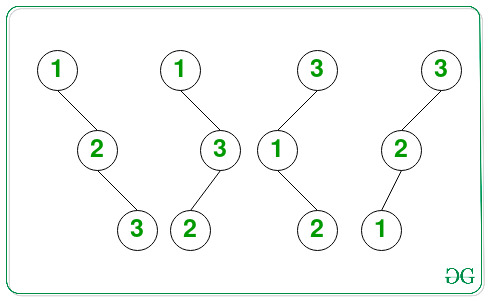Related Articles

# Number of Binary Search Trees of height H consisting of H+1 nodes

• Last Updated : 19 May, 2021

Given a positive integer H, the task is to find the number of possible Binary Search Trees of height H consisting of the first (H + 1) natural numbers as the node values. Since the count can be very large, print it to modulo 109 + 7.

Examples:

Input: H = 2
Output: 4
Explanation: All possible BSTs of height 2 consisting of 3 nodes are as follows:Therefore, the total number of BSTs possible is 4.

Input: H = 6
Output: 64

Approach: The given problem can be solved based on the following observations:

• Only (H + 1) nodes are can be used to form a Binary Tree of height H.
• Except for the root node, every node has two possibilities, i.e. either to be the left child or to be the right child.
• Considering T(H) to be the number of BST of height H, where T(0) = 1 and T(H) = 2 * T(H – 1).
• Solving the above recurrence relation, the value of T(H) is 2H.

Therefore, from the above observations, print the value of 2H as the total number of BSTs of height H consisting of the first (H + 1) natural numbers.

Below is the implementation of the above approach:

## C++

 `// C++ program for the above approach` `#include ``using` `namespace` `std;` `const` `int` `mod = 1000000007;` `// Function to calculate x^y``// modulo 1000000007 in O(log y)``int` `power(``long` `long` `x, unsigned ``int` `y)``{``    ``// Stores the value of x^y``    ``int` `res = 1;` `    ``// Update x if it exceeds mod``    ``x = x % mod;` `    ``// If x is divisible by mod``    ``if` `(x == 0)``        ``return` `0;` `    ``while` `(y > 0) {` `        ``// If y is odd, then``        ``// multiply x with result``        ``if` `(y & 1)``            ``res = (res * x) % mod;` `        ``// Divide y by 2``        ``y = y >> 1;` `        ``// Update the value of x``        ``x = (x * x) % mod;``    ``}` `    ``// Return the value of x^y``    ``return` `res;``}` `// Function to count the number of``// of BSTs of height H consisting``// of (H + 1) nodes``int` `CountBST(``int` `H)``{` `    ``return` `power(2, H);``}` `// Driver Code``int` `main()``{``    ``int` `H = 2;``    ``cout << CountBST(H);` `    ``return` `0;``}`

## Java

 `// Java program for the above approach``class` `GFG{``    ` `static` `int` `mod = ``1000000007``;` `// Function to calculate x^y``// modulo 1000000007 in O(log y)``static` `long` `power(``long` `x, ``int` `y)``{``    ` `    ``// Stores the value of x^y``    ``long` `res = ``1``;` `    ``// Update x if it exceeds mod``    ``x = x % mod;` `    ``// If x is divisible by mod``    ``if` `(x == ``0``)``        ``return` `0``;` `    ``while` `(y > ``0``)``    ``{``        ` `        ``// If y is odd, then``        ``// multiply x with result``        ``if` `((y & ``1``) == ``1``)``            ``res = (res * x) % mod;` `        ``// Divide y by 2``        ``y = y >> ``1``;` `        ``// Update the value of x``        ``x = (x * x) % mod;``    ``}` `    ``// Return the value of x^y``    ``return` `res;``}` `// Function to count the number of``// of BSTs of height H consisting``// of (H + 1) nodes``static` `long` `CountBST(``int` `H)``{``    ``return` `power(``2``, H);``}` `// Driver code``public` `static` `void` `main(String[] args)``{``    ``int` `H = ``2``;``    ` `    ``System.out.print(CountBST(H));``}``}` `// This code is contributed by abhinavjain194`

## Python3

 `# Python3 program for the above approach` `# Function to calculate x^y``# modulo 1000000007 in O(log y)``def` `power(x, y):``    ` `    ``mod ``=` `1000000007``    ` `    ``# Stores the value of x^y``    ``res ``=` `1` `    ``# Update x if it exceeds mod``    ``x ``=` `x ``%` `mod` `    ``# If x is divisible by mod``    ``if` `(x ``=``=` `0``):``        ``return` `0``        ` `    ``while` `(y > ``0``):``        ` `        ``# If y is odd, then``        ``# multiply x with result``        ``if` `(y & ``1``):``            ``res ``=` `(res ``*` `x) ``%` `mod` `        ``# Divide y by 2``        ``y ``=` `y >> ``1` `        ``# Update the value of x``        ``x ``=` `(x ``*` `x) ``%` `mod``    ` `    ``# Return the value of x^y``    ``return` `res` `# Function to count the number of``# of BSTs of height H consisting``# of (H + 1) nodes``def` `CountBST(H):``    ` `    ``return` `power(``2``, H)` `# Driver Code``H ``=` `2` `print``(CountBST(H))` `# This code is contributed by rohitsingh07052`

## C#

 `// C# program for the above approach``using` `System;` `class` `GFG{``    ` `static` `int` `mod = 1000000007;` `// Function to calculate x^y``// modulo 1000000007 in O(log y)``static` `long` `power(``long` `x, ``int` `y)``{``    ` `    ``// Stores the value of x^y``    ``long` `res = 1;` `    ``// Update x if it exceeds mod``    ``x = x % mod;` `    ``// If x is divisible by mod``    ``if` `(x == 0)``        ``return` `0;` `    ``while` `(y > 0)``    ``{``        ` `        ``// If y is odd, then``        ``// multiply x with result``        ``if` `((y & 1) == 1)``            ``res = (res * x) % mod;` `        ``// Divide y by 2``        ``y = y >> 1;` `        ``// Update the value of x``        ``x = (x * x) % mod;``    ``}` `    ``// Return the value of x^y``    ``return` `res;``}` `// Function to count the number of``// of BSTs of height H consisting``// of (H + 1) nodes``static` `long` `CountBST(``int` `H)``{``    ` `    ``return` `power(2, H);``}` `// Driver code``static` `void` `Main()``{``    ``int` `H = 2;``    ` `    ``Console.Write(CountBST(H));``}``}` `// This code is contributed by abhinavjain194`

## Javascript

 ``
Output:
`4`

Time Complexity: O(log2H)
Auxiliary Space: O(1)

Attention reader! Don’t stop learning now. Get hold of all the important DSA concepts with the DSA Self Paced Course at a student-friendly price and become industry ready.  To complete your preparation from learning a language to DS Algo and many more,  please refer Complete Interview Preparation Course.

In case you wish to attend live classes with experts, please refer DSA Live Classes for Working Professionals and Competitive Programming Live for Students.

My Personal Notes arrow_drop_up